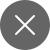# Complete Post-Mortem of a Confusion Matrix

5+

## What is the confusion matrix?

Most of us have heard the word Confusion matrix in Machine learning for classification problems. The confusion matrix is a table (a combination of rows and columns) that is used to evaluate machine learning classification problems for a given set of test data.  It is the N X N matrix, where N denotes the number of target classes in a given dataset.

For example –  for 2 target classes in the dataset, the matrix is of 2*2 table, for  3 target classes, the matrix is 3*3 tables. It shows the errors in the model’s performance in the form of a matrix, that’s why it is also known as an error matrix.

The matrix does a comparison between the actual values and predicted values by the model. This helps us to conclude how good our model is performing and what kind of errors it is making. Predicted values are those values that are predicted by our model and actual values are the true (provided) values for given inputs.

For binary classification,  the confusion matrix has a 2X2 matrix with 4 values as shown in the image.

I know you must be worried about the terms given in the confusion matrix. Let me simplify these terms for you.

We can see that the rows of the matrix represent predicted values and the columns represent actual values. But, hold on! What’s TP, FP, FN, TN here? Remember, these are the important terms in a confusion matrix.

### True Positive(TP)

True Positive is simply the case where the actual value as well as the predicted value are true. For instance, the person has been diagnosed with heart disease and the model also predicted that the person has heart disease.

### True Negative(TN)

True Negative is simply the case where the actual value and predicted value both are false. For instance, the person hasn’t been diagnosed with heart disease and the model also predicted that the person does not have heart disease.

### False Positive(FP)

False Positive is simply the case where the actual value is false but the predicted value is true. For instance, the person has not been diagnosed with heart disease and the model predicted that the person has heart disease. This is also known as a Type 1 error.

### False Negative(FN)

False Negative is just the case where the actual value is true but the predicted value is false. For instance, the person has been diagnosed with heart disease and the model predicted that the person does not have heart disease. This is also referred to as a Type 2  error.

All these things are fine but why do we need a confusion matrix? We have got other methods to test the performance of a classification problem than why confusion matrix. Let me clear this as well.

### Need for a Confusion Matrix

Accuracy(classification) =  correct predictions/total predictions

The main issue with classification accuracy is that it doesn’t provide much clarity regarding the performance of our model. The two major cases where you may face problems are –

• Suppose you want to predict if someone is diagnosed with cancer or not. There are 800 points for the negative class and 5 for the positive class.  This is an example of an imbalanced dataset. Let’s say the model is giving an accuracy of 85% i.e., it’s(model) trying to say I can predict diagnosed people 85% of the time. But in contrast, it’s predicting people who are not diagnosed with 85% accuracy and giving results for diagnosed people.
• When you’re having three or more classes, it is sometimes difficult to say for which class your model is doing good predictions and also the classes it’s neglecting.

### Derivations using Confusion Matrix

We can derive various useful terms, like accuracy, precision, recall, f-score, etc. using the confusion matrix.

Accuracy –  It determines how often the model is predicting the correct output. It is calculated as the ratio of several correct predictions done by the classifier to a total number of predictions.

Accuracy = correct predictions/total predictions

where correct predictions = TP+FP and, total predictions= TP+FP+TN+FN

Precision – This metric shows how likely the prediction of a positive class is correct.

Precision = TP/(TP+FP)

RecallThis metric shows what number of actual positive cases were predicted by our model.

Recall = TP/(TP+FN)

F-score – Usually, when we attempt to increase the precision, recall goes down and vice-versa. Here comes the role off-score, which captures both things in one picture.

F-score can be calculated by the given formula. It is maximum if the recall is equal to the precision.

F-score = 2*recall*precision/(recall+precision)

## Conclusion

I guess now the confusion matrix is not confusing for you anymore. I hope this article has helped you a lot to interpret and understand the confusion matrix. If you have liked the article, do upvote the article for some motivation.5+

### 2 Responses

1. December 14, 2020

[…] forget to check the confusion matrix and random forest […]

0
2. December 20, 2020

[…] check about – confusion matrix and cross-entropy […]

0